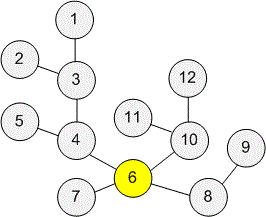Problems

# Find the centroid

Time limit 1 second
Memory limit 128 MiB

Find any centroid in the tree.

## Input data

The first line contains one integer n(1 ≤ n ≤ 2 · 10^5) — the number of vertices. Each of the following n − 1 lines contains two integers v_i and u_i(1 ≤ v_i , u_i ≤ n) - vertices connected with an edge.

It is guaranteed that this graph is a tree.

## Output data

Print the number of the vertex that is the centroid. If there are several centroids, print any one.## Examples

Input example #1
12
1 3
2 3
3 4
4 5
4 6
6 7
6 10
10 11
10 12
6 8
8 9

Output example #1
6